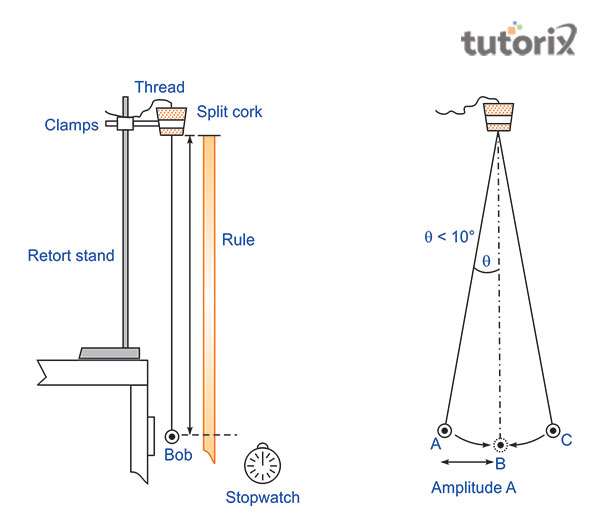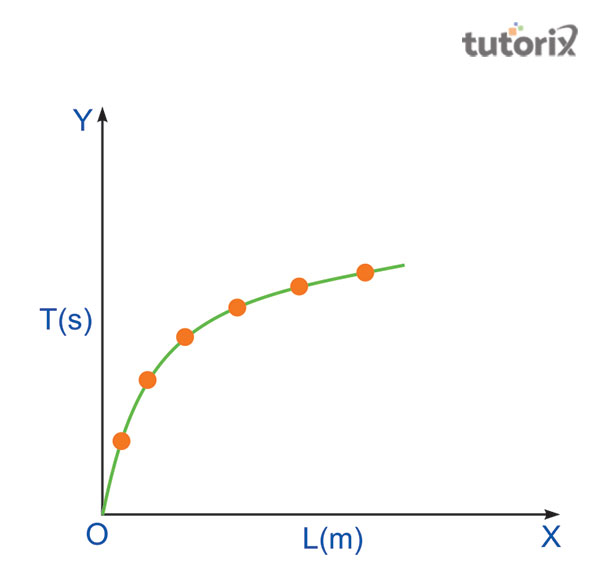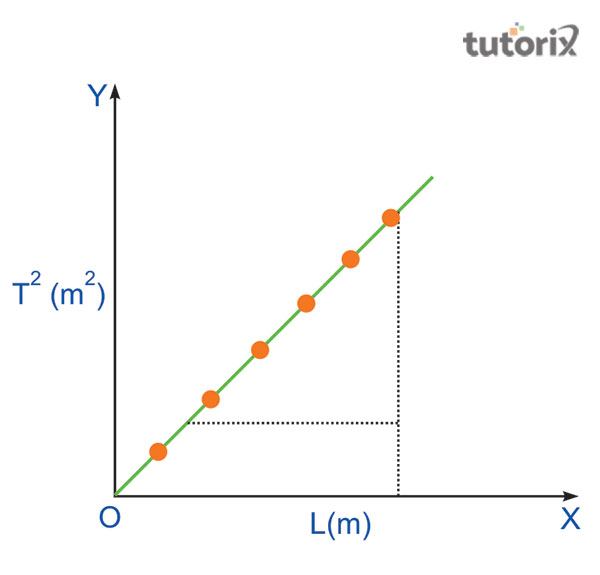# To find effective length of seconds pendulum using graph

The effective length of the seconds’ pendulum is the main experiment here. The experiment will be shown by using a graph and the L-T and L-T helps in the calculation of the simple pendulum.

## Aim

The main aim here is that with the application of a simple pendulum the plotting of L-T2 is done and it is applied to find the second pendulum’s effective length.

## Apparatus and Material Required

There is a need for some materials as well as apparatus for the finding of effective length. These are considered the most important materials for this examination. The materials are- split cork, clamp stand, Stopwatch, Long and strong cotton thread, Pencil Eraser, Meter scale, Graph Paper, and Heavy metallic spherical bob with a hook. These all are the materials that are needed for this application process.

## Principle

It is seen that the finding of the effective length of the seconds pendulum with the application of graph have some principles of it. The principle says that the simple pendulum exhibits Simple Harmonic Motion (SHM) as an acceleration of the pendulum bob. It is done because pendulum bob is directly proportional to the displacement from the mean position. It is always directed toward the displacement and here the period (T) of a simple pendulum is shown by using its relation.

## Procedure

• The procedure of the experiment goes through some steps and the very first step of it is that one has to place the clamp stand on the table.
• After that, the hook should have to be attached to the pendulum bob to one end of the string of a length of 150 cm in length (Cbselabs, 2022). T
• he other end of the string should have to be placed through two half-pieces of a split cork.
• Then one has to clamp the split cork tightly towards the stand so that the cork is placed at the right angle to the line of OA. in this experiment it will have to be taken care that the bob hangs vertically and let it hang behind the table’s edge so that it can freely oscillate.

In the figure below, one has to measure the effective length of the simple pendulum. Then the displacement of the bob will have to be done at an angle of not more than 15 degrees from that5 of the vertical position of OA.

In the next step, gently release it and if it is noticed that the stand seems shaky then a heavyweight will have to be placed on the base of it (Simo et al. 2019).

Some factors will have to be noticed as the bob does spin about its own axis. It does not move up-down during the time of oscillation. Another factor that will have to notice like the bob does not revolve in an elliptic path around its mean position. After keeping the pendulum oscillating, one has to wait for a few minutes.Figure 1: Effective Length of Pendulum

After that, when the oscillations are complete, start the stopwatch for the next step. The bob will cross the mean position after counting the oscillations 1, 2, 3. One should have to stop the stopwatch at the count of n oscillations for a better result in this matter. The repetition of the observation sometimes can help to notice down te the same n number of oscillations (Elkinany et al. 2020). The change of the pendulum’s length by about 10 cm can help in finding the time. The observations will be better if the use of tabular form is done and with the significant figures and proper unit.

## Observation Table

sl no. String's length from the top of the bob to the point of suspension Effective length, L = (l+r+e) Number of oscillations counted, n Time for n oscillations t (s) Time period T (= t/n)
cm m (i) (ii) (iii) Mean t (s)

Table 1: Time period calculation

## Plotting GraphFigure 2: Plotting Graph (L-T)Figure 3: Plotting Graph (L-T2)

(i) L vs T Graph

The above table helps in recording a plot between L versus T. Here L is taken on the x-axis and T is taken on the y-axis. It is shown that the graph is a curve and it is a part of the parabola as per figure 1.

(ii) L vs. T2 Graph

The observation table also helps in recording the plot of the graph between L versus T2. Here L is on the x-axis and T2 on the y-axis. Figure 2 shows that the graph presents a straight line that passes through the origin.

## Result

The result of the experiment is that the graph of L versus T is curved and it is convex upwards. The 2nd figure shows graph L vs. T2 creates a straight line. Here the effective length of the second’s pendulum is measured through cm.

## Precautions

Some precautions need to be taken care of in this part of the experiment. Here the thread should be strong as well as extensible. The suspension point should have to be fixed by a rigid support. The bob should not be spun during vibration.

## Conclusion

The experiment is done for finding the effective length of seconds’ pendulum with the use of two graphs. Here the graphs are presented for discussing the matter with an easy effort. The use of the pendulum experiment helps in measuring ng the time very effectively. The tutorial helps in defining the matter in an easy way.

## FAQs

Q1. What is a simple pendulum?

Simple pendulum is a single isolated particle and it is suspended by inextensible string and weightless flexible. It is done with the help of frictionless support.

Q2. What is simple harmonic motion?

The magnitude of the acceleration can create a direct proportion relationship to the displacement. It is called simple harmonic motion and is shown from the mean position.